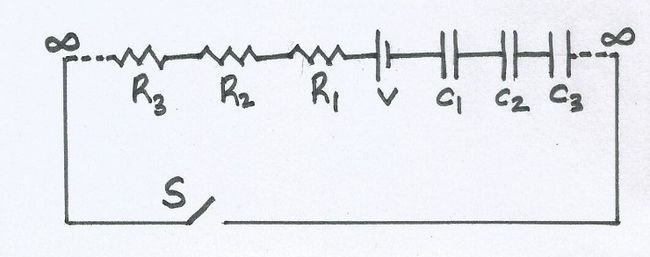# Infinite RC Circuit!In the circuit shown, we have infinitely many resistors with resistance of $R_1 = R, R_2 = \frac{R}{2}, R_3 = \frac{R}{4} , \ldots$. We also have infinitely many capacitors with capacitance of $C_1 = C, C_2 = 2C, C_3 = 4C ,\ldots$. At $t=0$ switch S is closed.

Find the ratio of potential drop (in volts) across capacitor $C_n$ and resistor $R_n$ at time $t=R_\text{eq}C_\text{eq}$. Take $e=2.718$.

×

Problem Loading...

Note Loading...

Set Loading...